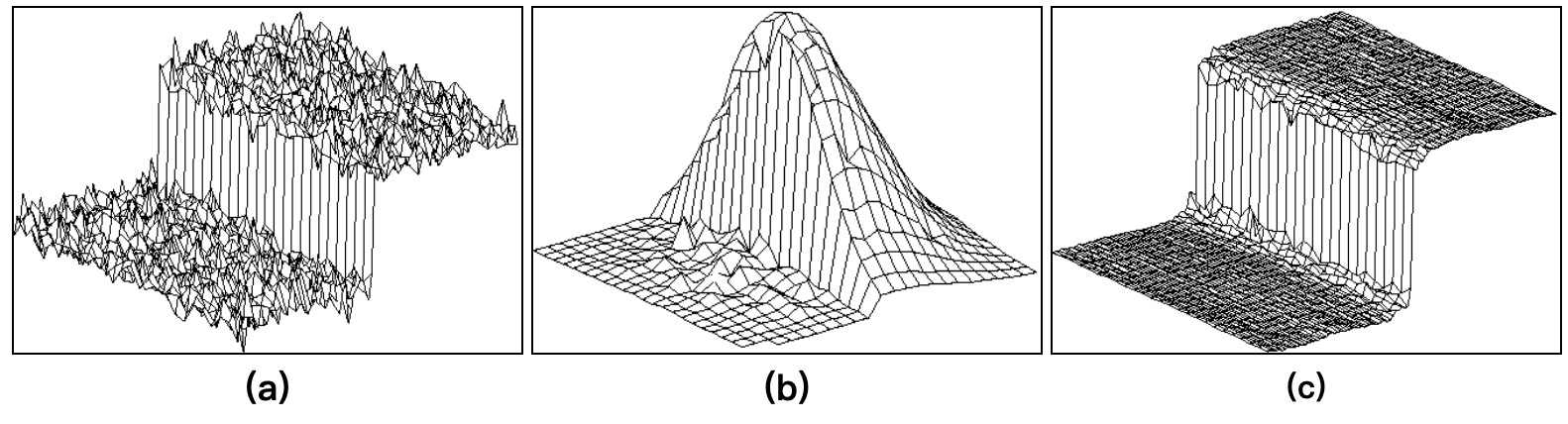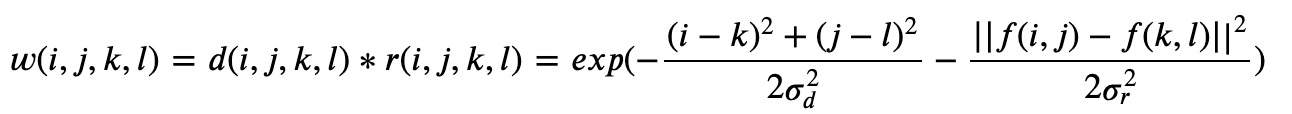# 图像处理: 双边滤波器

## 双边滤波器$$G(x,y) = \frac{1}{\sqrt {2 \pi \sigma ^2}} e ^{- \frac{(f(x,y) - f(x _c, y _c)) ^2}{2 \sigma ^2}}$$

$f(x,y)$ 表示当前点灰度值, $f(x _c, y_c)$ 表示滤波器中心灰度值.